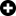# Dice Roller

Calculator

The Online Dice Roller can simulate the rolling of dice with 4, 6, 8, 10, 12, 20 and 100 sides, even several rolls at the same time. Choose the die you want to roll, then start rolling with the virtual dice.

## Dice Roller Online

Choose a die (or maybe several dice at the same time), then start rolling to see the result!?

Q&A

## How does the Online Dice Roller work?

The Online Dice Roller is able to roll dice with different numbers of sides, as well as to show the results. There are dice with four, six, eight, ten, twelve, twenty and one hundred sides, but only a die with 6 sides is considered to be a real die. This Online Dice Roller Simulator can simulate dice with different numbers of sides, which are used for example in role-playing games, so it may easily replace traditional dice. You may choose several virtual dice at the same time and roll them together (this excellent die roller made by bkis.)

## How many sides can a virtual die have?

There are d4, d6, d8, d10, d12, d20 and even d100 dices in this virtual dice collection, and you may use them in any combination. So, for example, you choose a virtual die with six sides and one with twenty sides, you roll them, and you get the results. The Online Dice Roller Simulator enables you to realize rolls that are very difficult or even impossible in real life, with real dice. For example, you can roll twenty dice with 12 sides each, and you can see the results one by one or as a sum. If you need a virtual dice with a different number of sides than the above ones, use the Random number generator that can simulate the roll of a dice with any number of sides.

## What are the odds of getting a given number when rolling with the virtual die?

Odds always depend on the number of sides as each side represents a possible outcome, a combination. For example, a die with 4 sides has 4 different outcomes, so the odds for each number are 0.25, that is 25 per cent.

## What are the odds of rolling an even number with a common die?

We generally refer to a die with 6 sides as a common die. In this case, for the calculus of probabilities, you have to consider the number of possible outcomes plus the number of outcomes that meet our criterion. A die of 6 sides has 6 possible outcomes. In our case, “good” results are even numbers – and there are 3 of them. All in all, the odds for rolling an even number with a common die are 0.5, that is 50 per cent. If you are interested in the calculus of probabilities, here you can learn more about the topic with the help of playful dice rolling probability examples.